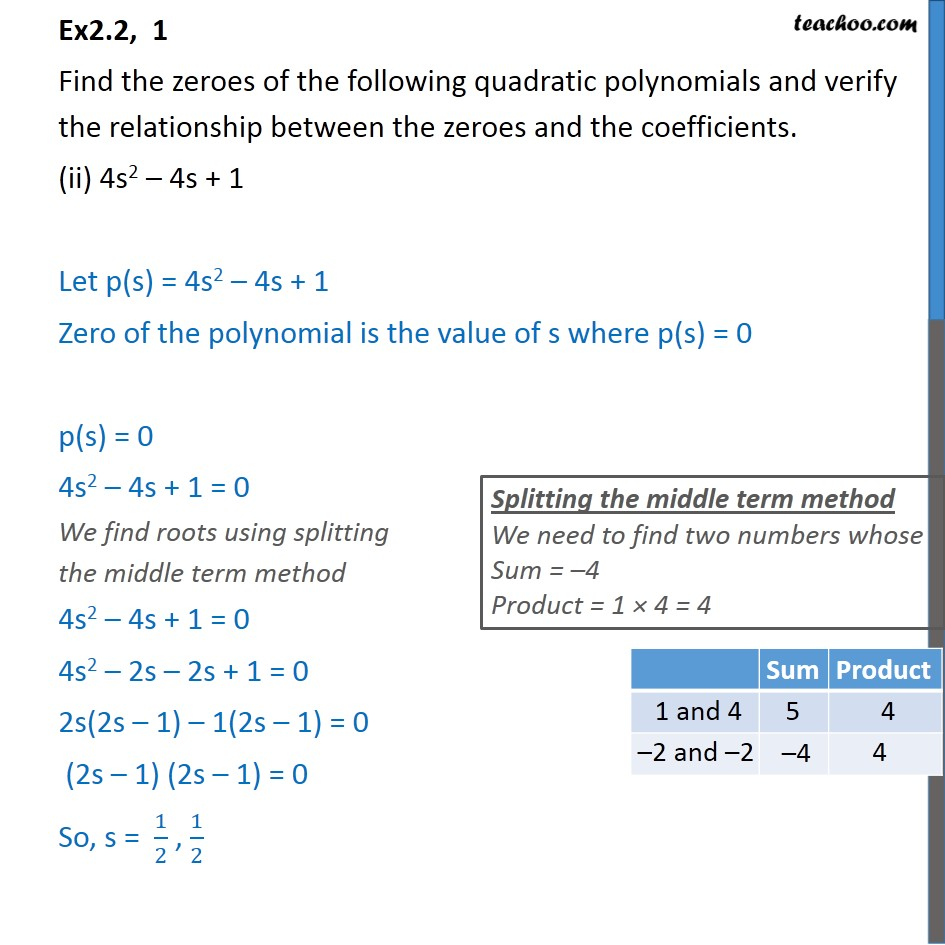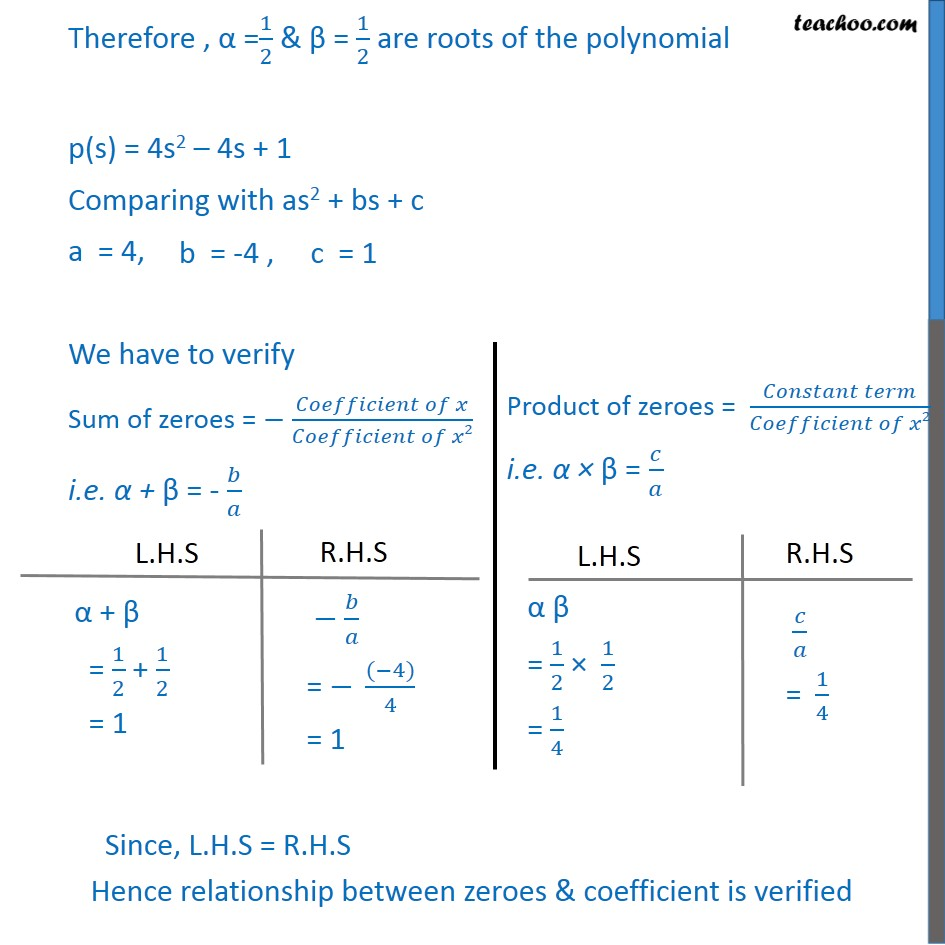1. Chapter 2 Class 10 Polynomials (Term 1)
2. Serial order wise
3. Ex 2.2

Transcript

Ex2.2, 1 Find the zeroes of the following quadratic polynomials and verify the relationship between the zeroes and the coefficients. (ii) 4s2 – 4s + 1 Let p(s) = 4s2 – 4s + 1 Zero of the polynomial is the value of s where p(s) = 0 p(s) = 0 4s2 – 4s + 1 = 0 We find roots using splitting the middle term method 4s2 – 4s + 1 = 0 4s2 – 2s – 2s + 1 = 0 2s(2s – 1) – 1(2s – 1) = 0 (2s – 1) (2s – 1) = 0 So, s = 1/2 , 1/2 Therefore , α =1/2 & β = 1/2 are roots of the polynomial p(s) = 4s2 – 4s + 1 Comparing with as2 + bs + c a = 4, We have to verify Sum of zeroes = − (𝐶𝑜𝑒𝑓𝑓𝑖𝑐𝑖𝑒𝑛𝑡 𝑜𝑓 𝑥)/(𝐶𝑜𝑒𝑓𝑓𝑖𝑐𝑖𝑒𝑛𝑡 𝑜𝑓 𝑥2) i.e. α + β = - 𝑏/𝑎